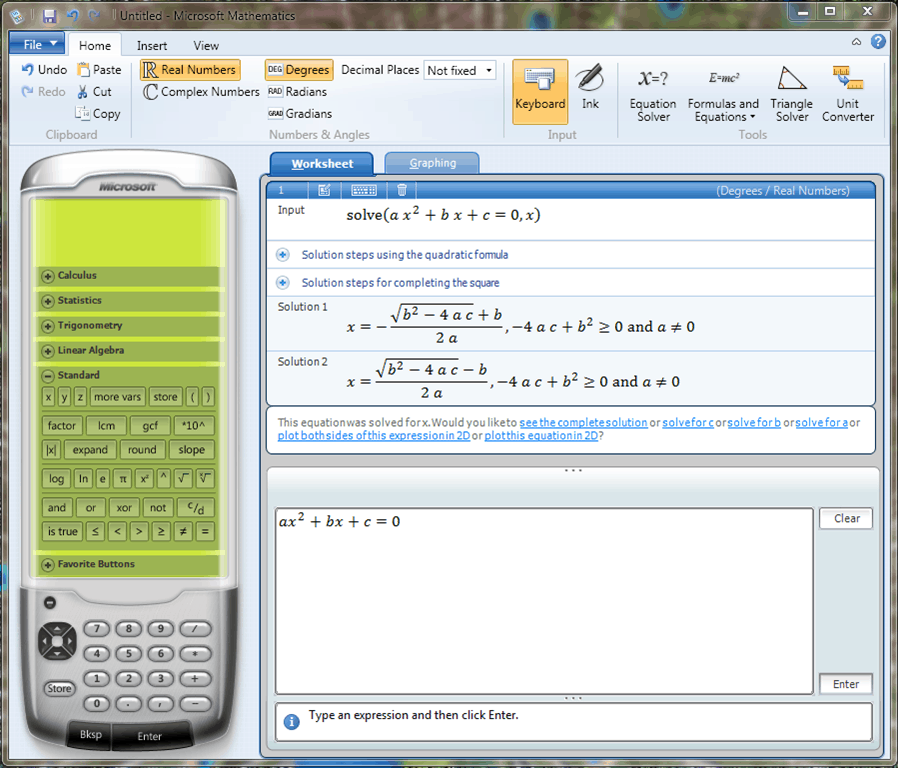Microsoft Mathematics is an application that provides a set of mathematical tools which will help students get their school work done quickly and easily. With Microsoft Mathematics, students can easily learn to solve equations step-by-step while gaining a better understanding of fundamental concepts in pre-algebra, algebra, trigonometry, physics, chemistry, and calculus.

Microsoft Mathematics includes a full-featured graphing calculator that’s designed to work just like a handheld calculator. Additional math tools help you evaluate triangles, convert from one system of units to another, and solve systems of equations.Mathematics 4.0 also comes with a friendly user interface designed with the popular ribbon interface. This differs from the Math add-in for Word and OneNote in that it has more of a learning focus than just providing equation support in documents.